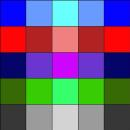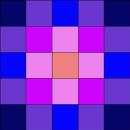#### You may also like### Diophantine N-tuples

Can you explain why a sequence of operations always gives you perfect squares?### DOTS Division

Take any pair of two digit numbers x=ab and y=cd where, without loss of generality, ab > cd . Form two 4 digit numbers r=abcd and s=cdab and calculate: {r^2 - s^2} /{x^2 - y^2}.### Sixational

The nth term of a sequence is given by the formula n^3 + 11n . Find the first four terms of the sequence given by this formula and the first term of the sequence which is bigger than one million. Prove that all terms of the sequence are divisible by 6.

# Attractive Tablecloths

##### Age 14 to 16Challenge Level

Attractive Tablecloths printable worksheet - tablecloths
Attractive Tablecloths printable worksheet - templates

Charlie has been designing square tablecloths for each weekday. He likes to use as many colours as he possibly can but insists that his tablecloths have some symmetry.

The $5$ by $5$ tablecloths below each satisfy a different symmetry rule.Monday's $5$ by $5$ tablecloth has just $1$ line of symmetry.    Design some square tablecloths of other odd by odd sizes with just $1$ line of symmetry. Check you agree that a $7$ by $7$ tablecloth can have at most 28 colours.   Can you find a way of working out the maximum number of different colours that can be used on an n by n tablecloth (where n is odd), following Monday's rule?Tuesday's $5$ by $5$ tablecloth has rotational symmetry of order $4$, and no lines of symmetry.     Design some square tablecloths of other odd by odd sizes with rotational symmetry of order $4$, and no lines of symmetry.   Check you agree that a $7$ by $7$ tablecloth can have at most 13 colours.   Can you find a way of working out the maximum number of different colours that can be used on an n by n tablecloth (where n is odd), following Tuesday's rule?Wednesday's $5$ by $5$ tablecloth has $2$ lines of symmetry (horizontal and vertical), and rotational symmetry of order $2$.     Design some square tablecloths of other odd by odd sizes with $2$ lines of symmetry, and rotational symmetry of order $2$.   Check you agree that a $7$ by $7$ tablecloth can have at most 16 colours.   Can you find a way of working out the maximum number of different colours that can be used on an n by n tablecloth (where n is odd), following Wednesday's rule?Thursday's $5$ by $5$ tablecloth has $2$ (diagonal) lines of symmetry and rotational symmetry of order $2$.     Design some square tablecloths of other odd by odd sizes with $2$ (diagonal) lines of symmetry and rotational symmetry of order $2$.   Check you agree that a $7$ by $7$ tablecloth can have at most 16 colours.   Can you find a way of working out the maximum number of different colours that can be used on an n by n tablecloth (where n is odd), following Thursday's rule?Friday's $5$ by $5$ tablecloth has $4$ lines of symmetry and rotational symmetry of order $4$.    Design some square tablecloths of other odd by odd sizes with $4$ lines of symmetry and rotational symmetry of order $4$.   Check you agree that a $7$ by $7$ tablecloth can have at most 10 colours.   Can you find a way of working out the maximum number of different colours that can be used on an n by n tablecloth (where n is odd), following Friday's rule?

EXTENSION
At weekends Charlie likes to use tablecloths with an even number of squares. Investigate the number of colours that are needed for different types of symmetric $n$ by $n$ tablecloths where $n$ is even.